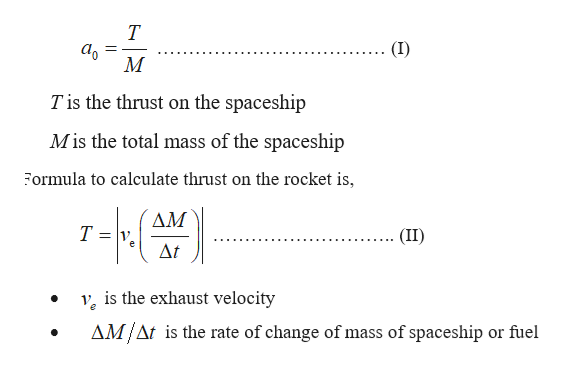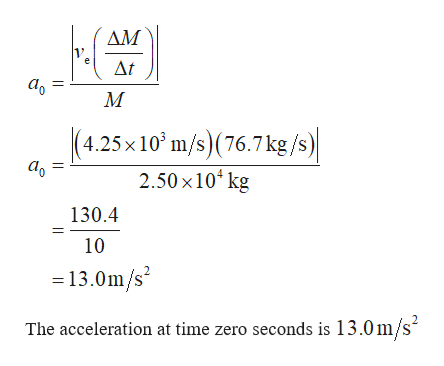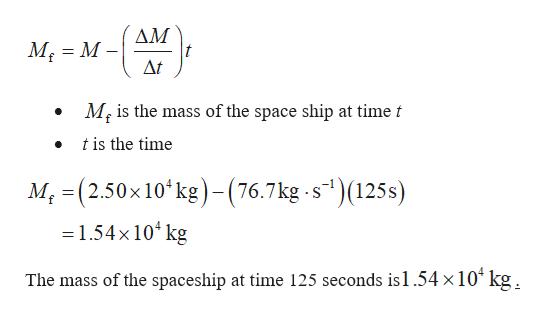# A spaceship at rest relative to a nearby star in interplanetaryspace has a total mass of 2.50 x 104 kg. Its engines fire at t = 0,steadily burning fuel at 76.7 kg/s with an exhaust speed of4.25 x 103 m/s. Calculate the spaceship’s (a) acceleration att = 0, (b) mass at t = 125 s, (c) acceleration at t = 125 s, and(d) speed at t = 125 s, relative to the same nearby star.

Question
7 views

A spaceship at rest relative to a nearby star in interplanetary
space has a total mass of 2.50 x 104 kg. Its engines fire at t = 0,
steadily burning fuel at 76.7 kg/s with an exhaust speed of
4.25 x 103 m/s. Calculate the spaceship’s (a) acceleration at
t = 0, (b) mass at t = 125 s, (c) acceleration at t = 125 s, and
(d) speed at t = 125 s, relative to the same nearby star.

check_circle

Step 1

a). Formula to calculate acceleration at time zero seconds is,help_outlineImage Transcriptionclose(I) M T is the thrust on the spaceship Mis the total mass of the spaceship Formula to calculate thrust on the rocket is, AM T = v, Δt (II) v, is the exhaust velocity AM/At is the rate of change of mass of spaceship or fuel fullscreen
Step 2

Use equation (II) in (I),help_outlineImage TranscriptioncloseДМ At м (4.25 x10° m/s)(76.7 kg /s) 2.50 x10ʻ kg 130.4 10 = 13.0m/s The acceleration at time zero seconds is 13.0m/s fullscreen
Step 3

b). Formula to calculate mass of the spa...help_outlineImage TranscriptioncloseДМ M, = M – Δ M, is the mass of the space ship at time t t is the time M; = (2.50x10ʻkg)- (76.7kg -s*)(125s) = 1.54x10* kg The mass of the spaceship at time 125 seconds is1.54 ×10 kg. fullscreen

### Want to see the full answer?

See Solution

#### Want to see this answer and more?

Solutions are written by subject experts who are available 24/7. Questions are typically answered within 1 hour.*

See Solution
*Response times may vary by subject and question.
Tagged in

### Physics